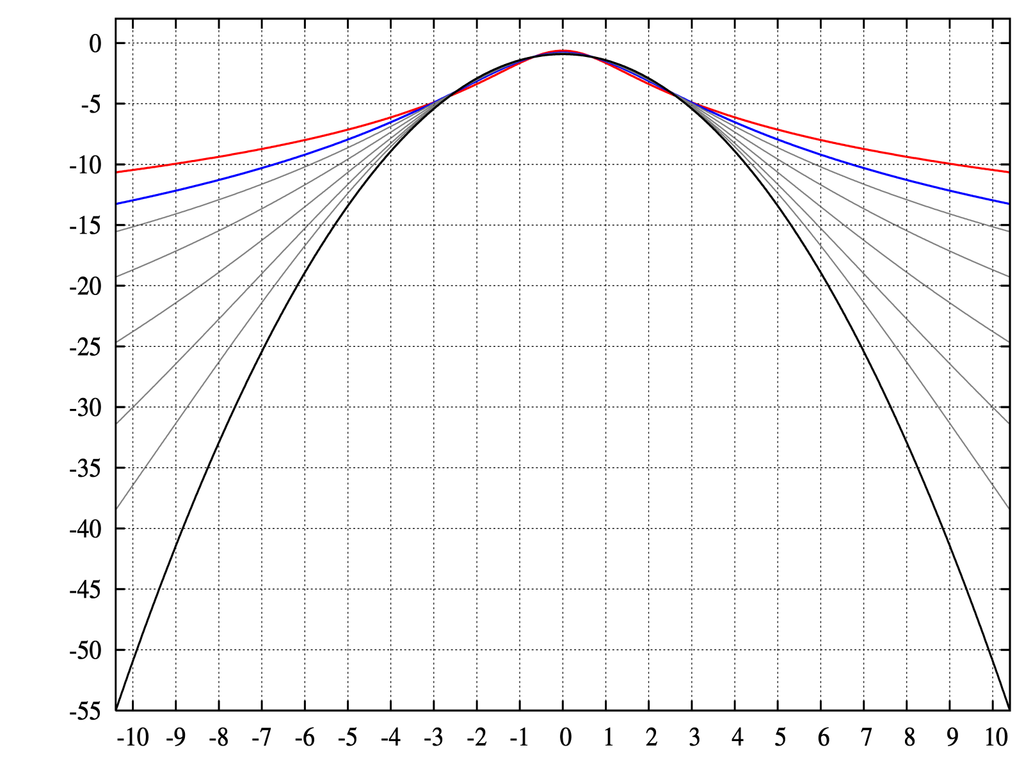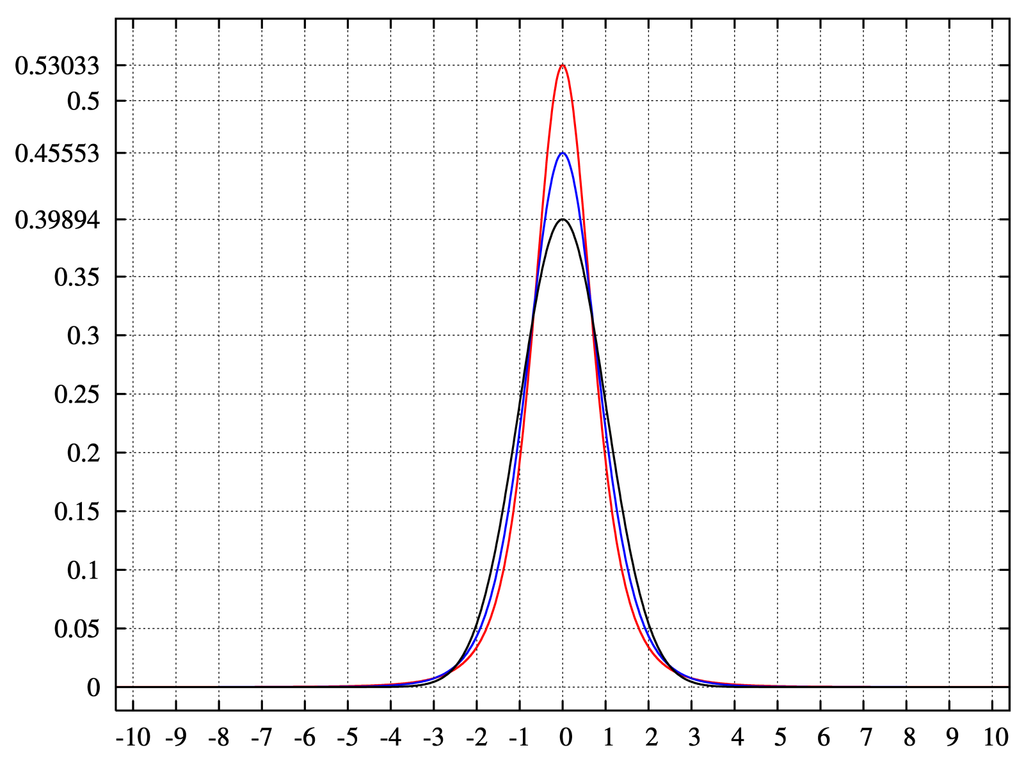# Kurtosis

## What is Kurtosis?

Kurtosis is the measurement of a functions "tailedness", often visually described by the sharpness of the peak values. Kurtosis is often explained in terms of the central peak. Higher values of it, indicate a higher, sharper peak; lower values indicate a lower, less distinct peak.

## How does Kurtosis work?The distribution on the left has a lower kurtosis value than the distribution on the right.

Source

Much like skewness is a measurement of a functions symmetry of distribution, kurtosis also describes characteristics of the function. In distribution analysis, kurtosis is referred to as the fourth moment. Each moment in a distribution is a property of the distribution. For example, the first ordinal moment is the distributions mean. The second moment is the variance. The third moment is the distribution's skewness. The fourth moment is a distribution's kurtosis. In a univariate normal distribution, for example, the kurtosis value is said to be 3. Often, kurtosis values are compared with that of the normal distribution as values less than 3 are said to be platykurtic, or "flat-topped." Alternatively, kurtosis values higher than 3 are said to be leptokurtic, usually appearing sharp at their peak value.